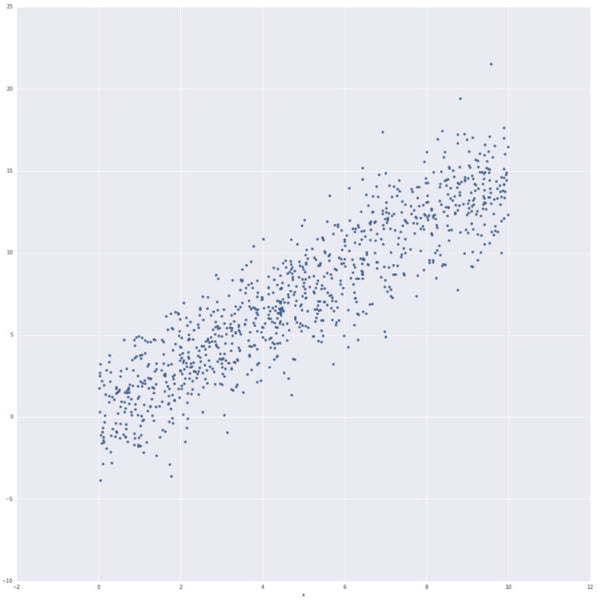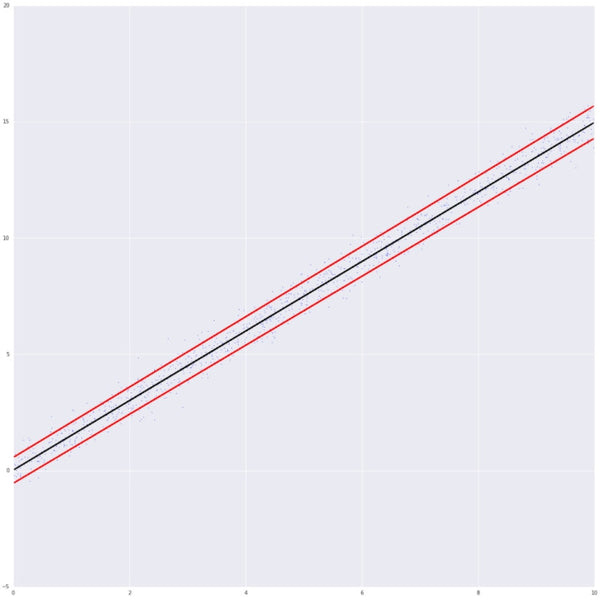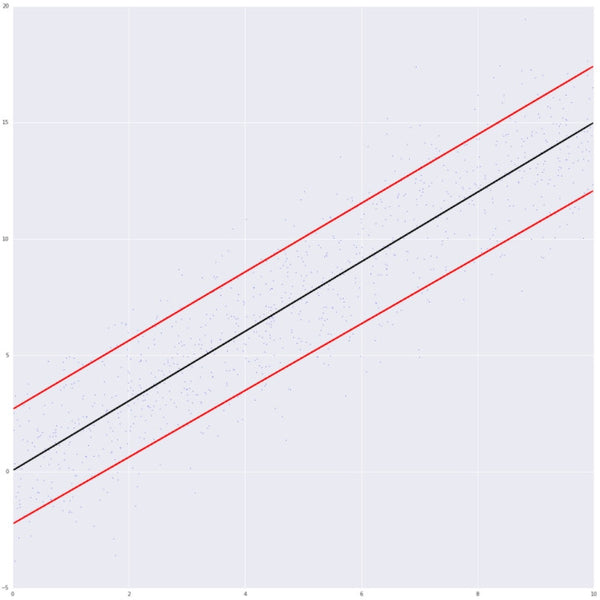Kyle Tate 07/11/2017

# How Shopify Capital Uses Quantile Regression To Help Merchants Succeed

6 minute read

Shopify Capital provides funding to help merchants on Shopify grow their businesses. But how does Shopify Capital award these merchant cash advances? In this post, I'll dive deep into the machine-learning technique our Risk-Algorithms team uses to decide eligibility for cash advances.

The exact features that go into the predictive model that powers Shopify Capital are secret, but I can share the key technique we use: quantile regression.

Quantile Regression

We determine eligibility for a cash advance chiefly around whether or not the amount we offer can be paid back through a percentage of sales in a reasonable time. To do so, we have to accurately predict what a merchant's future sales will look like: sounds a lot like regression to me!

The issue with regression is that it’s typically designed to fit the average of a distribution. In the context of Shopify Capital, fitting the average will not be sufficient: a prediction for the next 10 months of sales of $10,000 plus or minus$1,000 is a lot different than a prediction of $10,000 plus or minus$10,000. In the first case, we can have high confidence that a merchant will be able to pay back an advance of \$10,000 within 10 months by remitting 10% of their sales, whereas the certainty in the second case is much lower.

Let's dive deeper into what I mean when I say regression fits the average of a distribution and look at sample data for two simple linear regression problems:

If we run data.plot(x='x', y='y1', kind='scatter') and data.plot(x='x', y='y2', kind='scatter') we have the following distributions.

y1 distributiony2 distributionNow in the standard statistical setup for simple linear regression, we say that $$y$$ and $$x$$ are related as $$y \sim mx + b + e$$ where $$m$$ and $$b$$ are parameters that we want to find from the data and $$e$$ is a normally distributed random error. The regression problem is then to find $$m$$ and $$b$$ such that $$E(y | x) = mx + b$$, i.e. we solve for the mean of the conditional distribution. The solution for $$m$$ and $$b$$ turns out to be the values that minimize $$\sum (y - mx -b)^2 )$$ ( For a deeper dive on the theory underlying this post I highly recommend The Elements of Statistical Learning). Let's solve that numerically with python:

Running this I get (m1, b1) = (1.50, 0.02) and (m2,b2) = (1.50, 0.04), so even though the distribution for y2 is a lot wider, our regression for both will give the same results. That is because $$E(y_1 | x) = E(y_2 |x)$$ and both distributions have the same conditional mean, but they certainly don't have the same conditional variance. Ideally, we want to know the probability with which our prediction is greater than the true value. This will give us confidence in our prediction and quantile regression does exactly that.

As we saw above, in simple regression we are solving for $$E(y | x)$$ but in quantile regression we are making a prediction for $$Q_q(y | x)$$, i.e.: for a given quantile $$q$$ we want to make a set of predictions $$Q$$ so that $$q$$% of the true values are less than $$Q$$.

If we denote the residuals of our fit $$z = y - mx - b$$ then it turns out that instead of minimizing the sum of $$z^2$$, we need to minimize the sum of this function:

Notice that if $$q=0.5$$ (i.e. the median) then this is the same as minimizing the absolute value of the residuals. Let's do quantile regression for our problem above:

This gives me: (m1_q10, b1_q10) = (1.48, -0.55), (m1_q90, b1_q90) = (1.51, 0.56) for y1 and (m2_q10, b2_q10) = (1.43, -2.24) and (m2_q90, b2_q90) = (1.47, 2.68)) for y2. Plotting these gives us a better sense of the difference:

y1 distributiony2 distributionUsing this in practice
The theory above can be applied to real world situations such as judging the quality of white wine. It's all well and fine to predict the quality of a wine on average, but I'm very risk adverse: I only want to over-estimate a wine’s quality 10% of the time. Luckily quantile regression is going to let me do that:

So now we have our 0.1 quantile prediction of the test dataset white wine quality. We want to make sure that the true value in the test set is less than our quantile prediction only 10% of the time:

When I run this I get 0.092, which isn't bad! That means that if I use the prediction from this model I can be fairly certain that what I get will be as good or better than my prediction (i.e. I will over-estimate the quality of the wine 9.2% of the time). Perfect for those who don't want to be disappointed by their glass of wine. To see how we do this across the range of all quantiles we can run:

We can plot these to see that, across the quantile range, our method is giving us accurate probability predictions:Putting it all together

The white wine example above is a sample model. However, using the same quantile regression techniques we are able to offer merchant cash advances to Shopify merchants that make sense for their business. For merchants who are well established and have a proven track record of making sales, our model makes predictions for their future sales that have smaller error bands. For younger merchants that are just starting out, our model makes predictions that show a wider range of outcomes while ensuring that each advance offered has a high probability of being paid back in reasonable time. Those who start growing as a result of an early advance are then cycled back into the model triggering an update and allowing the model to offer them more next time.

Using quantile regression at Shopify we are able to make sure that our merchant cash advances can be offered to merchants regardless of whether they are new or established, while making sure that neither the merchant nor Shopify takes on too much risk. By using quantile regression, we have a better chance at seeing all our merchants succeed.